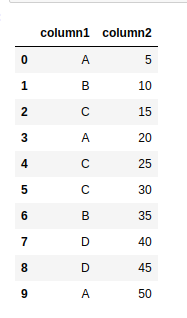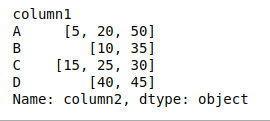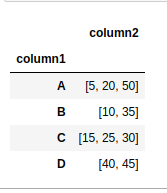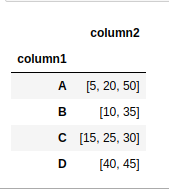Related Articles

# How to group dataframe rows into list in Pandas Groupby?

• Last Updated : 02 Feb, 2021

Suppose you have a pandas DataFrame consisting of 2 columns and we want to group these columns. In this article, we will discuss about the same. First, let;s create the dataframe.

## Python3

 `# importing pandas as pd``import` `pandas as pd`` ` ` ` `# Create the data frame``df ``=` `pd.DataFrame({``'column1'``: [``'A'``, ``'B'``, ``'C'``, ``'A'``, ``'C'``,``                               ``'C'``, ``'B'``, ``'D'``, ``'D'``, ``'A'``],``                   ``'column2'``: [``5``, ``10``, ``15``, ``20``, ``25``, ``30``, ``                             ``35``, ``40``, ``45``, ``50``]})`` ` `# Print the dataframe``df`

Output:Example #1: We can use groupby() method on column 1 and apply the method to apply a list on every group of pandas DataFrame.

## Python3

 `# importing pandas as pd``import` `pandas as pd`` ` ` ` `# Create the data frame``df ``=` `pd.DataFrame({``'column1'``: [``'A'``, ``'B'``, ``'C'``, ``'A'``, ``'C'``,``                               ``'C'``, ``'B'``, ``'D'``, ``'D'``, ``'A'``],``                   ``'column2'``: [``5``, ``10``, ``15``, ``20``, ``25``, ``30``, ``                             ``35``, ``40``, ``45``, ``50``]})`` ` ` ` `# Use groupby method and apply``# method on the dataframe``df ``=` `df.groupby(``'column1'``)[``'column2'``].``apply``(``list``)`` ` `# Print the dataframe again``df`

Output:Example #2: We can use groupby() method on column 1 and agg() method to apply aggregation, consisting of the lambda function, on every group of pandas DataFrame.

## Python3

 `# importing pandas as pd``import` `pandas as pd`` ` ` ` `# Create the dataframe``df ``=` `pd.DataFrame({``'column1'``: [``'A'``, ``'B'``, ``'C'``, ``'A'``, ``'C'``, ``                               ``'C'``, ``'B'``, ``'D'``, ``'D'``, ``'A'``], ``                   ``'column2'``: [``5``, ``10``, ``15``, ``20``, ``25``, ``30``,``                               ``35``, ``40``, ``45``, ``50``]})`` ` `# Use groupby method and agg method ``# with lambda function on the dataframe``df ``=` `df.groupby(``'column1'``).agg({``'column2'``: ``lambda` `x: ``list``(x)})`` ` `# Print the dataframe again``df`

Output:Example #3: We can use the groupby() method on column 1 and agg() method to apply the aggregation list, on every group of pandas DataFrame.

## Python3

 `# importing pandas as pd``import` `pandas as pd`` ` ` ` `# Create the data frame``df ``=` `pd.DataFrame({``'column1'``: [``'A'``, ``'B'``, ``'C'``, ``'A'``, ``'C'``,``                               ``'C'``, ``'B'``, ``'D'``, ``'D'``, ``'A'``],``                   ``'column2'``: [``5``, ``10``, ``15``, ``20``, ``25``, ``30``, ``                               ``35``, ``40``, ``45``, ``50``]})`` ` `# Use groupby method and agg method ``# with list as argument on the dataframe``df ``=` `df.groupby(``'column1'``).agg(``list``)`` ` `df`

Output:Example #4: We can use groupby() method on column 1 and agg() method by passing ‘pd.Series.tolist’ as an argument.

## Python3

 `# importing pandas as pd``import` `pandas as pd`` ` ` ` `# Create the data frame``df ``=` `pd.DataFrame({``'column1'``: [``'A'``, ``'B'``, ``'C'``, ``'A'``, ``'C'``, ``                               ``'C'``, ``'B'``, ``'D'``, ``'D'``, ``'A'``], ``                   ``'column2'``: [``5``, ``10``, ``15``, ``20``, ``25``, ``30``,``                               ``35``, ``40``, ``45``, ``50``]})`` ` `# Use groupby method and agg method with``# pd.Series.tolist as argument on the dataframe``df ``=` `df.groupby(``'column1'``).agg(pd.Series.tolist)`` ` `df`

Output:Attention geek! Strengthen your foundations with the Python Programming Foundation Course and learn the basics.

To begin with, your interview preparations Enhance your Data Structures concepts with the Python DS Course. And to begin with your Machine Learning Journey, join the Machine Learning – Basic Level Course

My Personal Notes arrow_drop_up